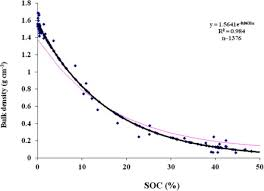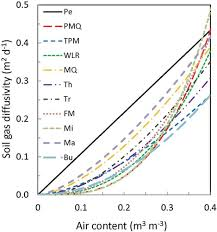## How to Calculate and Solve for Bulk Density | Soil Mechanics and FoundationThe image above represents bulk density.

To compute for bulk density, two essential parameters are needed and these parameters are Mass of the soil (m) and Volume of the soil (V).

The formula for calculating bulk density:

sb = m / V

Where:

sb = Bulk Density
m = Mass of the Soil
V = Volume of the Soil

Let’s solve an example;
Find the bulk density when the mass of the soil is 24 and the volume of the soil is 6.

This implies that;

m = Mass of the Soil = 24
V = Volume of the Soil = 6

sb = m / V
sb = 24 / 6
sb = 4

Therefore, the bulk density is 4.

Calculating the Mass of the Soil when the Bulk Density and the Volume of the Soil is Given.

m = sb x V

Where;

m = Mass of the Soil
sb = Bulk Density
V = Volume of the Soil

Let’s solve an example;
Find the mass of the soil when the bulk density is 10 and the volume of the soil is 4.

This implies that;

sb = Bulk Density = 10
V = Volume of the Soil = 4

m = sb x V
m = 10 x 4
m = 40

Therefore, the mass of the soil  is 40.

## How to Calculate and Solve for Air Content of the Soil | Soil Mechanics and FoundationThe image above represents air content of the soil.

To compute for air content of the soil, two essential parameters are needed and these parameters are Volume of the Air (Vaand Volume of the soil (V).

The formula for calculating air content of the soil:

A = Va / V

Where:

A = Air Content of the Soil
Va = Volume of the Air
V = Volume of the Soil

Let’s solve an example;
Find the air content of the soil when the volume of the air is 20 and the volume of the soil is 24.

This implies that;

Va = Volume of the Air = 20
V = Volume of the Soil = 24

A = Va / V
A = 20 / 24
A = 0.83

Therefore, the air content of the soil is 0.83.

Calculating the Volume of the Air when the Air Content of the Soil and the Volume of the Soil is Given.

Va = A x V

Where;

Va = Volume of the Air
A = Air Content of the Soil
V = Volume of the Soil

Let’s solve an example;
Find the volume of the air when the air content of the soil is 12 and the volume of the soil is 3.

This implies that;

A = Air Content of the Soil = 12
V = Volume of the Soil = 3

Va = A x V
Va = 12 x 3
Va = 36

Therefore, the volume of the air is 36.